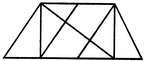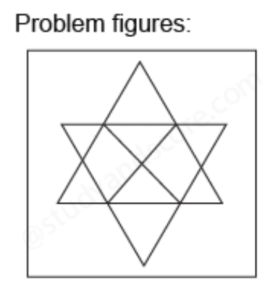Welcome to your Analytical Quiz 15

You have 50 green balls and 50 red ones and 2 containers. If you divide the balls into each container to maximize the probability of selecting a green ball randomly, what is the probability of getting a green ball (in %) equal to?Two pipes P and Q fill a tank in 14 and 12 minutes respectively, they are opened simultaneously. After how much time should pipe Q be closed so that the tank is full in 10 minutes?
Count the number of parallelograms in the given figure.Add description here!
The vast majority of pigeons are gray. What is the most logical conclusion?
3, 7, 25, 73, 173, ? Find the value of the question mark.
If the marked price of 30 commodities is equal to the selling price of 40 commodities, what is the percentage discount?